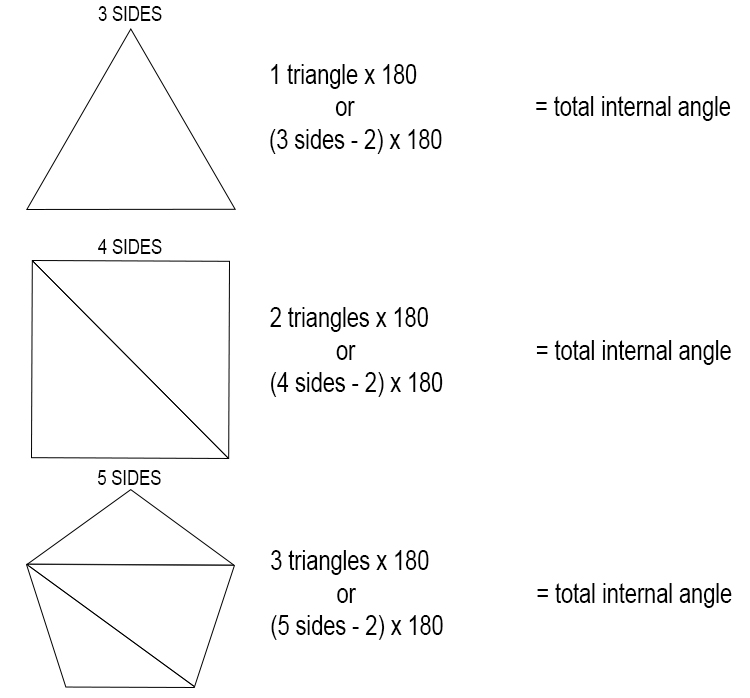# Sum of all internal angles of a polygon

To remember the formula for the sum of all internal angles of a polygon, you should always work out the first three polygons, i.e triangle, square and pentagon.Therefore, the sum of all the internal angles

=(Number  of  sides -2)times180

which is often shown as

=(n-2)times180

where n is the number of sides.